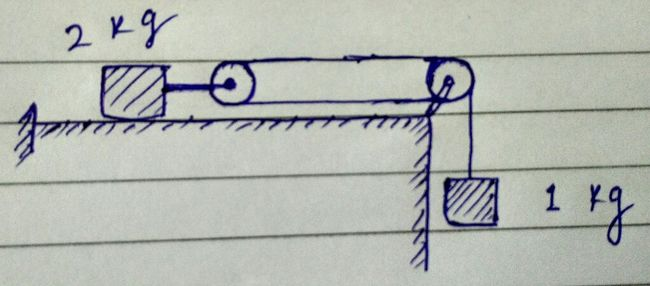# Constraint relation 03Consider the situation shown in figure. Both the pulleys and the string are light and all the surfaces are smooth. The tension in the string connecting block of mass 1 kg and pulley is $\dfrac ab$ where $a$ and $b$ are coprime positive integers. Find the value of $a-b$.

Take $g=10\text{ m/s}^2$.

×